## 10 May 2016

### Agler (5.5) Symbolic Logic: Syntax, Semantics, and Proof, "Additional Derivation Rules (PD+)", summary

by Corry Shores
[Search Blog Here. Index-tags are found on the bottom of the left column.]

[The following is summary. Boldface (except for metavariables) and bracketed commentary are my own. Please forgive my typos, as proofreading is incomplete. I highly recommend Agler’s excellent book. It is one of the best introductions to logic I have come across.]

Summary of

David W. Agler

Symbolic Logic: Syntax, Semantics, and Proof

Ch.5: Propositional Logic Derivations

Brief summary:

The set of 11 “intelim” propositional derivation rules, called PD, just by themselves can lead to lengthy proofs, so to them we add six more rules, to make a system called PD+. The following chart shows all of PD+, with the new ones being 13-17.

Summary

We previously discussed 11 “intelim” derivation rules in a system of propositional derivation called PD. With only these rules, making proofs can at times be cumbersome. So Agler adds six more rules, which in addition to the prior 11, make PD+.

5.5.1 Disjunctive Syllogism (DS)

We begin with the derivation rule called disjunctive syllogism (DS). [Let us first think semantically about disjunctions. We made this truth table evaluation.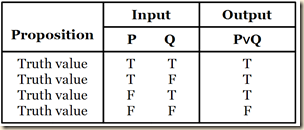The disjunction is false only when both disjuncts are false. But suppose that we begin by knowing that the disjunction as a whole is true. In our table, that limits us to the first three rows. And suppose further that we learn that one of the disjuncts is false, although we do not necessarily know the truth value of the other disjunct. So this limits us to the second and third row of the table. As we see, in both cases, whenever one disjunct is false, the other is true.] The way DS works is that if we have a disjunction and if we have also the negation of one of the disjuncts given on its own, then we can derive the other disjunct.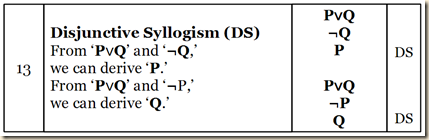Agler will now demonstrate the usefulness of adding DS to our other rules, and he will do so by showing that in certain cases, if we do not use it, the proof can get quite complicated. His example is:

P∨Q, ¬Q ⊢ P

(209)

As we can see, this involves a fairly complex proof, when we only use our PD rules.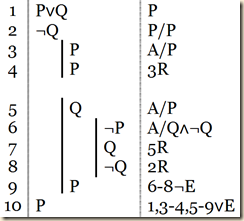[Now note that were we to use the DS rule, we have everything we need already in our two premises.] But with the DS rule at our disposal, here is the proof for the exact same argument.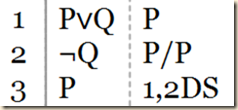Or consider this argument:

P↔(Q∨R), P, ¬Q ⊢ R

(Agler 210)

Using just PD we would proof it like this.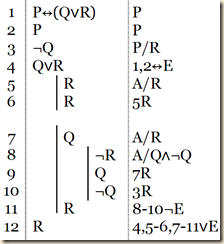But with the option of DS, we can solve it much more efficiently.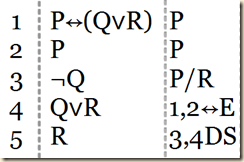(210)

We should be sure not to make the following mistake. We begin with a disjunction. We also have one of the disjuncts. Then we derive the other disjunction. This is wrong. The only way DS works is if we have the negation of one of the disjuncts (210).

5.5.2 Modus Tollens (MT)

Now we turn to the modus tollens (MT) derivation rule. Suppose we begin with a conditional, and we also have the negation of the consequent. We can then derive the negation of the antecedent. [Again see this first semantically from the truth tables.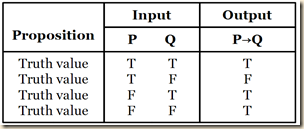First suppose that we know the whole conditional is true. That limits us to lines 1, 3, and 4. And suppose further that we also know that the consequent is false. This leaves us only with line 4, where the antecedent is false.]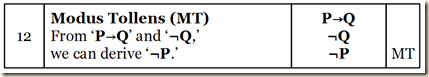Agler shows how MT can simply proofs. Consider first:

P→Q, ¬Q  ⊢ ¬P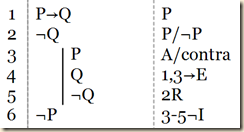(Agler 211)

However, we could skip steps 3-5 using MT.

We can see MT work even with more complex propositions as the parts of the conditionals, as in this case:

(P∧Z)→(Q∨Z), ¬(Q∨Z) ⊢ ¬(P∧Z)

(Agler 211)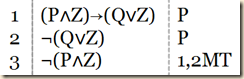(Agler 211)

5.5.3 Hypothetical Syllogism (HS)

Suppose we have two conditionals, and the consequent of one is the antecedent of the second. Using Hypothetical syllogism (HS) we can then derive a conditional with the antecedent of the first with the consequent of the second.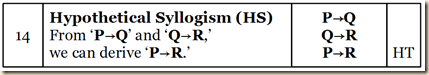(Agler 211)

We can use HS to simplify proofs for arguments with multiple conditionals [sharing middle terms]. Consider the following one:

P→Q, Q→R ⊢ P→R

(Agler 212)

As we can see, this can be solved with just one additional step beyond the premises, if we use HS. Agler shows the longer way, to illustrate its usefulness.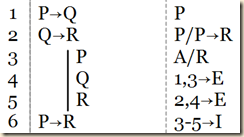(Agler 212)

5.5.4 Double Negation (DN)

The double negation derivation rule (DN) allows us to go from a doubly negated proposition to an unnegated form, and vice versa.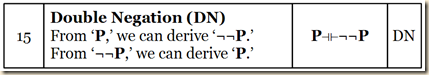This makes it an equivalence rule, and so it can be written:

P ⊣ ⊢ ¬¬P

which combines the following two derivation rules:

P ⊢ ¬¬P
¬¬P ⊢ P

(Agler 212)

One important thing to note about DN is that it can apply not only to complex propositions on a whole but also to any constituent proposition in a complex one.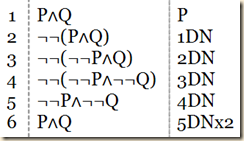(Agler 212-213)

The elimination of a double negation only works when there are two negations side-by-side, without a parenthesis or other operators or terms between them. And when we introduce double negations, they likewise must be placed side-by-side: “the rule demands that a single proposition can be replaced with a proposition that is doubly negated or a single doubly negated proposition can be replaced by removing its double negation” (213).

5.5.5 De Morgan’s Laws (DeM)

De Morgan’s Laws (DeM) will allow us to deal “with negated conjunctions and negated disjunctions” “by introducing an equivalence rule that allows for expressing every negated conjunction in terms of a disjunction and every negated disjunction in terms of a conjunction” (213).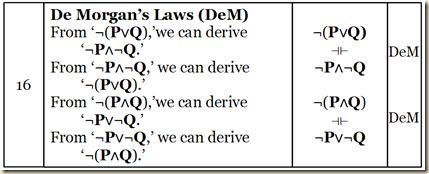De Morgan's Laws will enable us to shorten many proofs. Agler has us consider the proof for

¬(P∧Q) ⊢ ¬P∨¬Q

(Agler 213)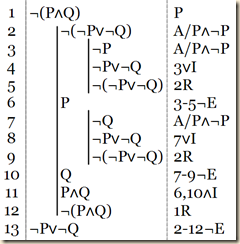With the help of DeM, we can simplify this to one step.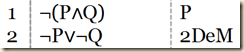(Agler 214)

DeM is also useful for making derivations that allow for elimination rules. Consider:

¬(P∨Q) ⊢ ¬P

We cannot apply any elimination rules directly to ¬(P∨Q). But if we make a DeM derivation, we can.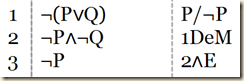(Agler 214)

5.5.6 Implication (IMP)

Although our derivation rules will not allow us to derive something directly from a negated biconditional, we do have a rule, Implication (IMP) that will allow a derivation from a negated conditional. It is an equivalence rule that allows us to derive a negated conjunction from a conditional and vice versa.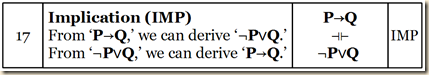Agler illustrates by having us consider the following.

P→Q ⊢ ¬P∨Q

(Agler 215)

Agler will show how without IMP, the proof for this can be quite long. [Recall the strategic rule SA#4(∨): If the conclusion is a disjunction, assume the negation of the whole disjunction, derive a contradiction, and then use ‘¬I’ or ‘¬E.’ (Agler 199)] Since in this proof we have a disjunction, we should follows strategic assumption rule 4 and assume the negation of the entire disjunction, with the aim of deriving a contradiction. We see below how lengthy that process can be.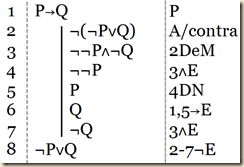[Note, in my version of the text, the justification for line 4 reads “1∧E”.] But all these derivations can be achieved in just one step using IMP.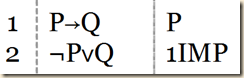(Agler 215)

Agler then compares two ways to make the proof for:

¬P∨Q ⊢ P→Q

(Agler 215)

[Recall strategic assumption rule SA#2(→): If the conclusion is a conditional, assume the antecedent, derive the consequent, and use ‘→I.’] As we can see, the conclusion is a conditional, P→Q, so in accordance with SA#2, we should assume P and try to derive Q. As we can see, we can do so using disjunctive syllogism.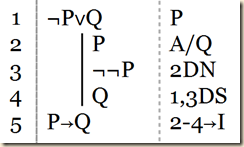(Agler 215)

But we can solve it in one step using IMP [like above] (215).

Agler says that IMP also helps with proofs involving negated conditionals. He has us consider:

¬(P→Q) ⊢ ¬Q

(Agler 216)

Let us set it up first.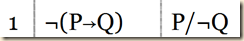We first use our new rule IMP.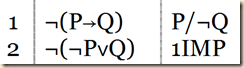We see we have a negated disjunction. At this point, we do not have  any way to apply an elimination rule, which we will need to derive our atomic goal proposition ¬Q. But since it is a negated conjunction,we could use De Morgan’s laws.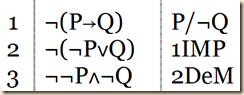We now have a conjunction containing our goal proposition. So we can use conjunction elimination.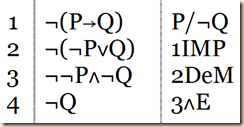(Agler 216)

Agler, David. Symbolic Logic: Syntax, Semantics, and Proof. New York: Rowman & Littlefield, 2013.